Next: Weight Up: Computing with Classical Modular Previous: Compute Every Elliptic Curve   Contents

# Find all elliptic curves of conductor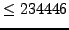Andrei Jorza, Jen Balakrishnan, and I verified that the Stein-Watkins tables
   http://modular.math.washington.edu/Tables/ecdb/

are complete for prime conductors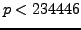. This proved that the smallest conductor of a rank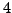elliptic curve is not prime. Is the smallest conductor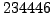? To find out, one has to compute every elliptic curve (up to isogeny) of conductor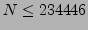. Cremona has computed every curve of conductor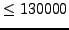, and much more about each curve (e.g., pretty much everything we know how to compute about a curve).

Problem 4.2.1   Determine all elliptic curves over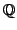of conductor. By determine'' this could be man finding just the first few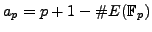for each curve, not the actual equation.

The Stein-Watkins tables

   http://modular.math.washington.edu/Tables/ecdb/

contains a substantial chunk'' of the curves of conductor. Challenge 4.2.1 amounts to finding the number (and some info about) the curves that are missing from Stein-Watkins in the range of conductors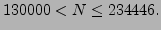Next: Weight Up: Computing with Classical Modular Previous: Compute Every Elliptic Curve   Contents
William Stein 2006-10-20